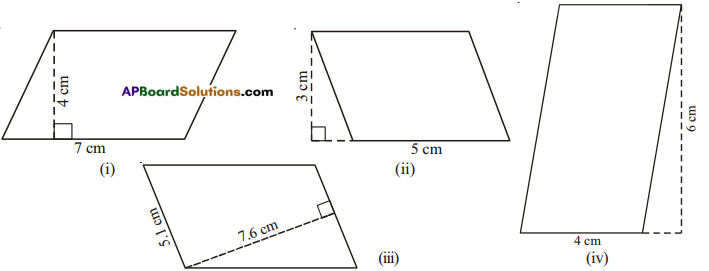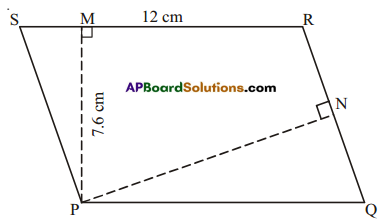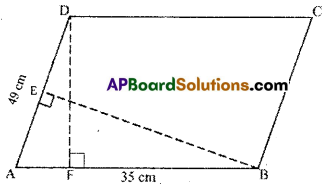# HSSlive: Plus One & Plus Two Notes & Solutions for Kerala State Board

## AP Board Class 7 Maths Chapter 13 Area and Perimeter Ex 2 Textbook Solutions PDF: Download Andhra Pradesh Board STD 7th Maths Chapter 13 Area and Perimeter Ex 2 Book AnswersAP Board Class 7 Maths Chapter 13 Area and Perimeter Ex 2 Textbook Solutions PDF: Download Andhra Pradesh Board STD 7th Maths Chapter 13 Area and Perimeter Ex 2 Book Answers

## Andhra Pradesh State Board Class 7th Maths Chapter 13 Area and Perimeter Ex 2 Books Solutions

 Board AP Board Materials Textbook Solutions/Guide Format DOC/PDF Class 7th Subject Maths Chapters Maths Chapter 13 Area and Perimeter Ex 2 Provider Hsslive

2. Click on the Andhra Pradesh Board Class 7th Maths Chapter 13 Area and Perimeter Ex 2 Answers.
3. Look for your Andhra Pradesh Board STD 7th Maths Chapter 13 Area and Perimeter Ex 2 Textbooks PDF.
4. Now download or read the Andhra Pradesh Board Class 7th Maths Chapter 13 Area and Perimeter Ex 2 Textbook Solutions for PDF Free.

## AP Board Class 7th Maths Chapter 13 Area and Perimeter Ex 2 Textbooks Solutions with Answer PDF Download

Find below the list of all AP Board Class 7th Maths Chapter 13 Area and Perimeter Ex 2 Textbook Solutions for PDF’s for you to download and prepare for the upcoming exams:

Question 1.
Find the area of the each of the following parallelograms.Solution:

 Fig Base Height A = Base x Height (i) 7 4 7 x 4 = 28cm2 (ii) 5 3 5 x 3 = 15 cm2 (iii) 5.1 7.6 5.1 x 7.6 = 38.76 cm2 (iv) 4 6 4 x 6 = 24cm2

Question 2.
PQRS is a parallelogram. PM is the height from P to SR⎯⎯⎯⎯⎯⎯⎯ and PN is the height from P to QR⎯⎯⎯⎯⎯⎯⎯⎯. If SR = 12cm and PM = 7.6 cm.
(i) Find the area of the parallelogram PQRS
(ii) Find PN, if QR = 8 cm.
Solution:i) Given:SR = 12cm
PM = 7.6cm
∴ Area = base × height
= 12 × 7.6 = 91.2 cm2

ii) Also area = base × height
91.2 = QR × PN
91.2 = 8 × PN
∴ PN = 91.28 = 11.4cm

Question 3.
DF and BE are the height on sides AB and AD respectively in parallelogram ABCD. Ifthe area of the parallelogram is 1470 cm2, AB = 35 cm and AD = 49 cm, find the length of BE and DF.Solution:
Given : AB = 35 cm, AD = 49 cm
area of 🖾ABCD = 1470 cm2
Area of a parallelogram = base × height
AB × DF = area of 🖾ABCD
35 × DF = 1470
DF = 147035 = 42cm
Also AD × BE = 1470
49 × BE = 1470
∴ BE= 147049 = 30cm

Question 4.
The height of a parallelogram is one third of its base. If the area of the parallelogram is 192cm2, find its height and base.
Solution:
Area of the parallelogram = 192 cm2
Area of parallelogram = base × height = 192 cm2 (given)
Let the base of the parallelogram be x.
height = 𝑥3
Area = base × height = x x 𝑥3=𝑥23 = 192
⇒ x2 = 192 × 3 = 576
x = 576‾‾‾‾√ = 24
∴ base = 24 cm
height = 𝑥3=243 = 8cm.

Question 5.
In a parallelogram the base and height are is in the ratio of 5:2. If the area of the parallelogram is 360m2, find its base and height.
Solution:
Let the base of the parallelogram be 5x and height of the parallelogram be 2x.
Area = height x base = 5x × 2x = 10x2 = 360 cm2 (given)
10x2 = 360
x2 = 36010 = 36
x = √36 = 6
base = 5x = 5(6) = 30 cm
height = 2x = 2(6) = 12 cm

Question 6.
A square and a parallelogram have the same area. If a side of the square is 40m and the height of the parallelogram is 20m, find the base of the parallelogram.
Solution:
Given: Side of a square = 40m
Height of a parallelogram = 20m
Area of parallelogram = Area of the square
base × height side × side
base × 20 40 × 40
∴ base = 40×4020 = 80 m.

## Andhra Pradesh Board Class 7th Maths Chapter 13 Area and Perimeter Ex 2 Textbooks for Exam Preparations

Andhra Pradesh Board Class 7th Maths Chapter 13 Area and Perimeter Ex 2 Textbook Solutions can be of great help in your Andhra Pradesh Board Class 7th Maths Chapter 13 Area and Perimeter Ex 2 exam preparation. The AP Board STD 7th Maths Chapter 13 Area and Perimeter Ex 2 Textbooks study material, used with the English medium textbooks, can help you complete the entire Class 7th Maths Chapter 13 Area and Perimeter Ex 2 Books State Board syllabus with maximum efficiency.

## FAQs Regarding Andhra Pradesh Board Class 7th Maths Chapter 13 Area and Perimeter Ex 2 Textbook Solutions

#### Can we get a Andhra Pradesh State Board Book PDF for all Classes?

Yes you can get Andhra Pradesh Board Text Book PDF for all classes using the links provided in the above article.

## Important Terms

Andhra Pradesh Board Class 7th Maths Chapter 13 Area and Perimeter Ex 2, AP Board Class 7th Maths Chapter 13 Area and Perimeter Ex 2 Textbooks, Andhra Pradesh State Board Class 7th Maths Chapter 13 Area and Perimeter Ex 2, Andhra Pradesh State Board Class 7th Maths Chapter 13 Area and Perimeter Ex 2 Textbook solutions, AP Board Class 7th Maths Chapter 13 Area and Perimeter Ex 2 Textbooks Solutions, Andhra Pradesh Board STD 7th Maths Chapter 13 Area and Perimeter Ex 2, AP Board STD 7th Maths Chapter 13 Area and Perimeter Ex 2 Textbooks, Andhra Pradesh State Board STD 7th Maths Chapter 13 Area and Perimeter Ex 2, Andhra Pradesh State Board STD 7th Maths Chapter 13 Area and Perimeter Ex 2 Textbook solutions, AP Board STD 7th Maths Chapter 13 Area and Perimeter Ex 2 Textbooks Solutions,
Share: